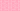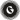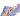PricingResources

# Return on Assets: Definition, Formula and ExamplesWritten by

## Return on Assets Explained

One of the most important questions you can ask yourself when looking at a business – particularly if you’re thinking of working with or investing in that business – is how profitable it is. At face value this may seem like a fairly simple question to answer; the income a business generates minus the expenses it incurs will leave you with a figure which represents the profit generated by a business.

Calculating the return on assets, however, delivers a far more accurate picture of how efficiently a business is making use of the assets it owns. Once it has been calculated, the return on assets is expressed as a percentage, and the higher the percentage is the better it is for the business in question. Calculating the return on assets of a number of different companies of comparable size working in the same sector makes it possible to see which of those businesses is the most well run.

## The return on assets formula

While comparing profits to revenue gives an idea of how successfully a business is operating, making a comparison between those profits and the resources that had to be exploited to generate the profits gives a much fuller picture, and the good news is that the return on assets formula is, in basic terms, actually fairly simple.

The return on assets figure is calculated by taking the net income of a business and dividing that figure by the total assets of the company. The calculation can be set out as follows:

## The details used for calculating the return on assets

The good news is that if the company in question is a public company, the figures required to generate the return on assets percentage will be freely available via publicly reported income statements and balance sheets, which are generally published annually, quarterly or monthly.

In order to gain a return on assets figure that reflects the situation over a prolonged period, rather than simply being a snapshot of the position on the date the figures were published, it is necessary to take an average of figures over that period. This is particularly useful in light of the fact that the assets a company holds can fluctuate from time to time when items such as large pieces of equipment or properties are bought and sold. In this calculation, the figure used for the assets will be an average, so the formula will be expressed as:

### An example of a return on assets calculation

Perhaps the easiest way of explaining how return on assets calculations work is to give a real world example, in this case using the Exxon Mobil Corporation:

• In 2016 the total assets of Exxon were valued at \$330 billion

• In 2017 the total assets of Exxon were valued at \$349 billion

By adding these two figures together and dividing by two we arrive at the average assets over the period 2016/2017 of \$339.5 billion. In 2017, the net income of Exxon – meaning the profits after expenses – was \$19.7 billion. Calculating the return on assets for Exxon over this period therefore means dividing \$19.7 billion by \$339.5 billion, which gives a figure of 5.8%. This means that for every dollar of assets held by Exxon in 2017 the company generated 5.8 cents in profit. In comparison, the same figures for two other major oil companies in 2017 were as follows:

• ROA for the Chevron Corporation =3.57%

• ROA for British Petroleum =1.26%

## We can help

The more efficiently a business is run, the higher the return on assets percentage will be, and the latest payments technology from GoCardless can play a part in making that happen. We can help to keep cash flowing through your business helping with ad hoc payments or recurring payments.Contact sales

Sales

Support